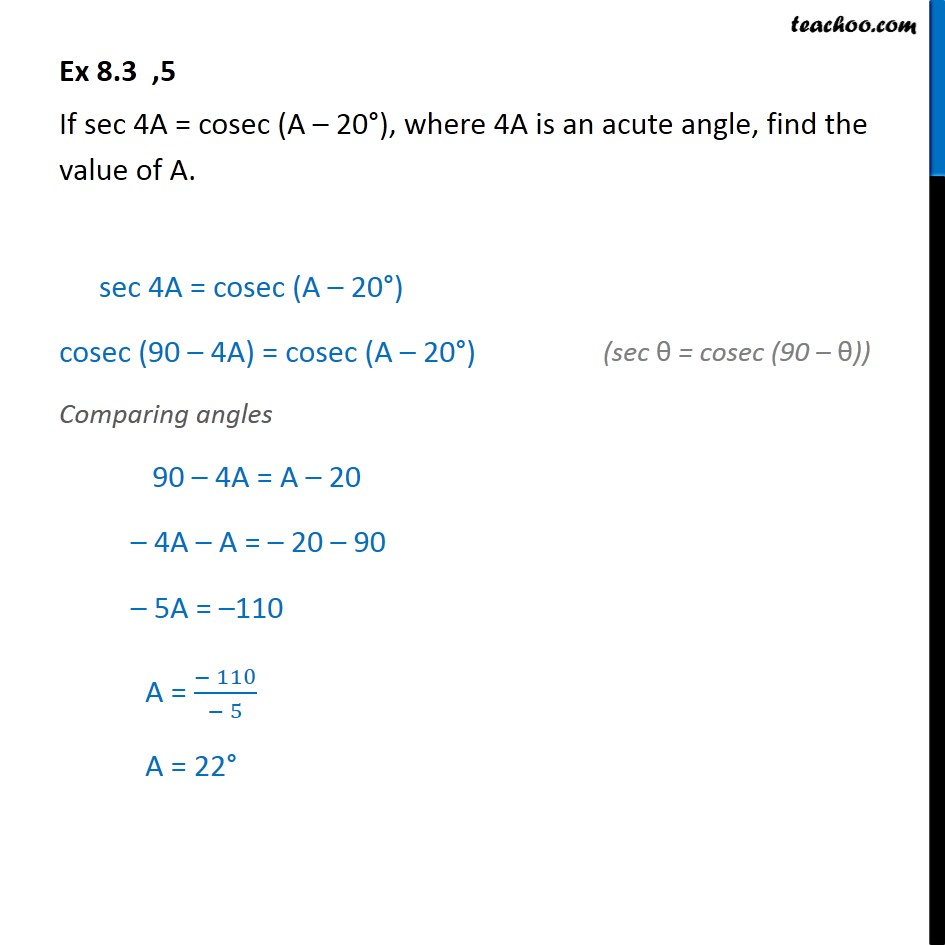1. Class 10
2. Important Questions for Exam - Class 10
3. Chapter 8 Class 10 Introduction to Trignometry

Transcript

Ex 8.3 ,5 If sec 4A = cosec (A – 20°), where 4A is an acute angle, find the value of A. sec 4A = cosec (A – 20°) cosec (90 – 4A) = cosec (A – 20°) Comparing angles 90 – 4A = A – 20 – 4A – A = – 20 – 90 – 5A = –110 A = (− 110)/(− 5) A = 22°

Chapter 8 Class 10 Introduction to Trignometry

Class 10
Important Questions for Exam - Class 10